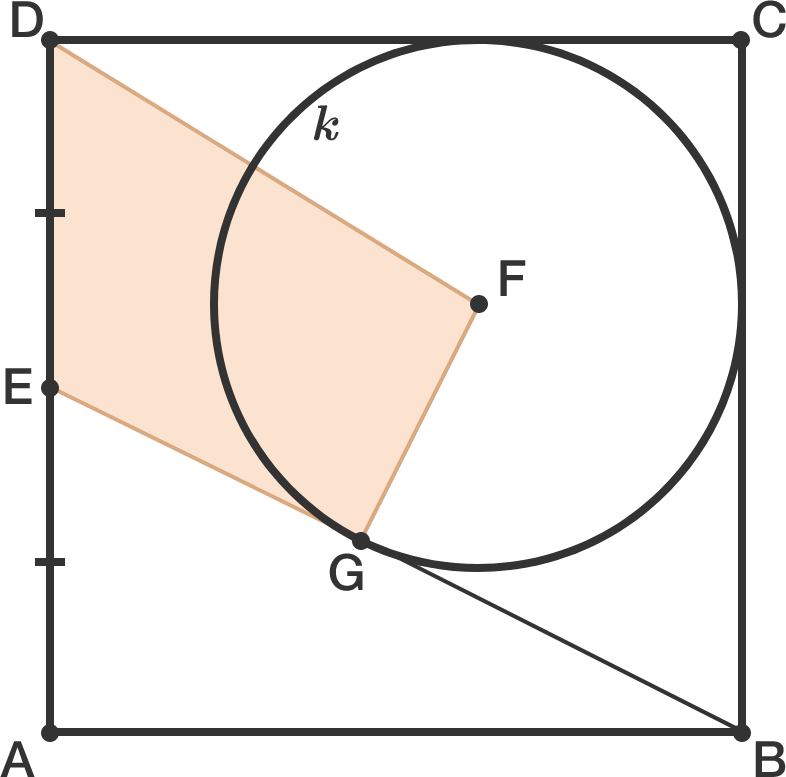# Strange quadrilateral

Geometry Level 2

$ABCD$ is a square with side length 1. $E$ divides side $AD$ into two equal parts. Circle $k$ centered at point $F$ is tangential to sides $BC$, $CD$ and segment $BE$.

If $G$ is the point where circle $k$ touches $BE$, find the area of quadrilateral $EGFD$.×

Problem Loading...

Note Loading...

Set Loading...